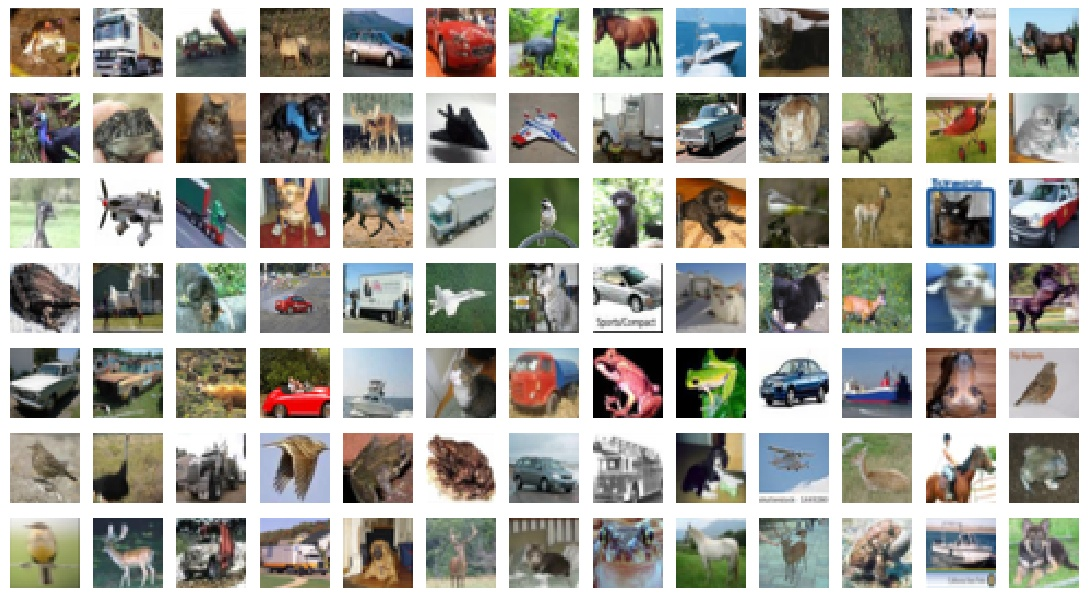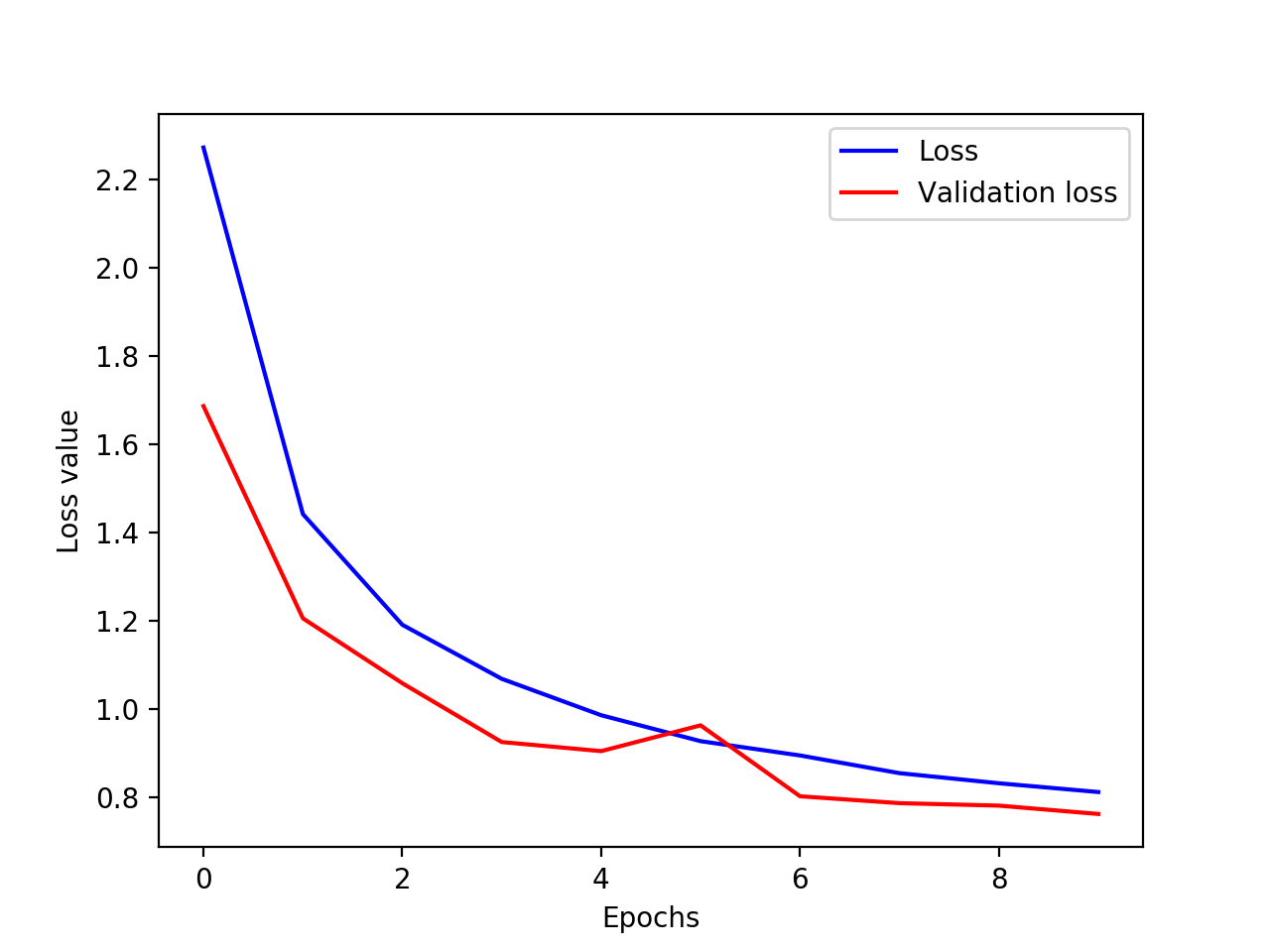# Image classification with Convolutional Neural Networks

Convolutional Neural Networks are the state of art approach to classify images. In this post I will show you what Convolutional neural networks (CNNs) are and how you can use them for image classification. Together we will apply them to the famous CIFAR-10 data-set and classify all the images in 10 different categories.

What you will learn:

• What CNNs are
• How you can implement CNNs with Keras and Python in no time
• What CIFAR-10 data-set is and how you can use it with Keras

## The Problem: CIFAR-10

The CIFAR-10 data set servers as a the perfect example here. This data set consists of colored images with a size of 32×32. Each image needs to be classified into one of the 10 categories. Take  a look at the image below to see samples from the data set.Normally loading and preprocessing data sets is a tedious task. But we are lucky: Keras provides us with an easy way to load the CIFAR-10 data set. The following code loads the data set for us. Since the labels are provided as numbers in the range 0-10, we need to convert them to a one-hot vector representation (line 6-7).

```from keras.datasets import cifar10
from keras.utils import to_categorical

(x_train, y_label_train), (x_test, y_label_test) = cifar10.load_data()

y_train = to_categorical(y_label_train, 10)
y_test = to_categorical(y_label_test, 10)```

## Convolutional neural network basics

For our image classification problem we will focus on 2d convolutions. However, CNNs can be applied in very different scenarios and with different dimensions (1d and 3d). For a more detailed explanation see my post on Convolutional Neural Networks in Depth [will be linked soon].

Convolutional neural networks (CNNs) are a special version of the Multi-layer Perceptron (MLP). However, there is a key difference. MLPs have one big weight matrix and each weight is applied to exactly one pixel. Convolutional Neural Networks instead consist of one or more smaller filter matrices. These matrices are applied to small areas of the images while sliding over the whole image. This allows the network to recognize structures in the image independent of the position. At the same time it reduces the size of the weight matrices.

The size of the filters can be chosen freely, but smaller filter values around 3×3 are the most common. While bigger filters can capture more complex structures, smaller filters keep more information in the image and have a smaller weight matrix to train. Thus its common to use small filter sizes combined with a deep network, as we do in the following model.

## Model

For our problem I choose a rather simple model. The model only consists of 5 convolutional layers and a Global Average Pooling layer for the output.

It is a rather new idea to use an Global Average Pooling layer directly on top of the last convolutional layer. In traditional architectures the output layers are a number of fully connected layers. However, these layers are hard to train and prone to overfitting. For that reason newer architectures like the SqueezeNet rely on a Global Average Pooling layer. This layer takes each channel of the previous convolutional layer and builds the average of all values in that channel. Thus, the number of channels in the last convolution needs to match the number of categories in the output.

Apart from that the architecture is pretty much standard. The convolutional layers are combined into blocks, followed by a Max Pooling and Dropout layer. The Dropout layer randomly throws away some of the output values of the previous layer (at a rate of 25% in our case). This is a very common and highly efficient method to prevent overfitting. As an activation function a linear rectifier unit (ReLu) is used in all cases, which allows us to learn non-linear functions.

In the following you can see the corresponding Keras code.

```from keras.models import Sequential
from keras.layers import Conv2D, Activation, GlobalAvgPool2D, MaxPooling2D, Dropout

model = Sequential()

## Training

Now that we have the data we can start training our model:

```model.compile('adam', 'categorical_crossentropy', metrics=['accuracy'])
model.fit(x_train, y_train, batch_size=100, epochs=10, validation_split=0.1)```

For this example I just use an Adam optimizer with default parameters. (In a future post I will discuss the benefits and downsides of the different optimizers. So stay tuned.) In addition I use the accuracy as a metric since the loss alone is harder to interpret. To decide if we are overfitting we use 10% of our training data as a validation set. The plot below shows the training and validation loss over the whole training phase.As you can see both the training-set and the validation-set loss are going down (with one exception at the 6. epoch). This shows us that the training is working and we are not overfitting. With longer training it is likely that the loss is going down even further, before the validation loss is going up again and we overfit on the training data. Now, let’s review our final result on the test set.

## Result

To get an accurate picture of our model, we need to evaluate it on the test-set:

`m.evaluate(x_test, y_test)`

For a training run with 10 epochs, the best result was 73.05% test-set accuracy. Giving the short training time that’s an impressive result. With a longer training, it’s likely that the accuracy will be even higher, but for demonstration purposes, I did not try longer training. However, the code is available on GitHub, feel free to download it and train over a longer period of time.

This site uses Akismet to reduce spam. Learn how your comment data is processed.